## Tuesday, September 4, 2012

### The Largest Shape That Can Fit

We are finding the dimensions of the largest object that can fit in another geometric shape.

Largest Circle in a Square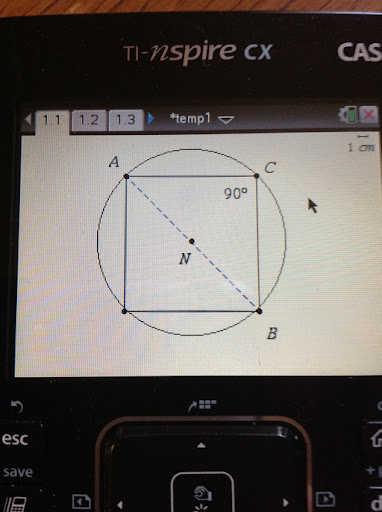Known: The radius, which is represented by line segment

AN
.
Goal: Find the dimension of one side of the square. Let the measure of the line segment AC be represent the measure of the side.

Let:
AB

represent the line segment which length is the diameter of the circle.

By the Pythagorean Theorem:

AC^2 + CB^2 = AB^2

Since AC = CB and AB = 2 AN,

2 AC^2 = (2 AN)^2
AC^2 = 2 AN^2
AC = AN √2

Take the positive root, as the negative root has negligible meaning.

The measure of the square's side is the radius times √2. (s = r √2)

Largest Circle in a Square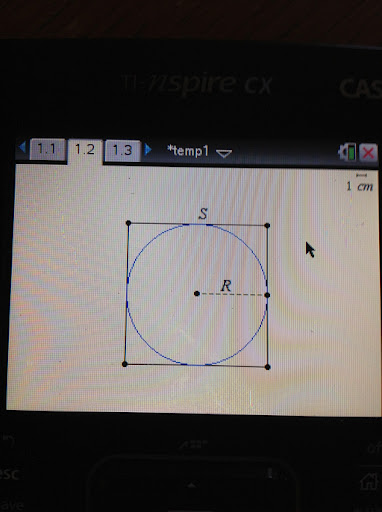Known: Radius of the circle, R.
Goal: Find the length of a side of the square, S.

Observe that the largest circle will fit when it's center is in the square's center. As the picture above indicates, some of the edge of the circle touches the square.

Therefore, S = 2 R

Largest Triangle in a Square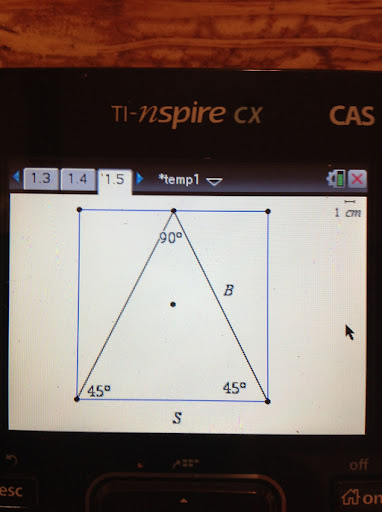Known: A square with each side measuring S.
Goal: The dimensions of the largest triangle.

If we want the largest triangle to fit in the square, it would make sense to make one of the sides to be of length S. It would also make sense to have the vertex of the triangle's two other sides at the other side of the square. This can be accomplished by a 45°-45°-90° triangle, not an equilateral triangle. (See the diagram above)

To solve for B, use trigonometry.

Observe that sin 45° = cos 45° = √2 / 2

Then:

√2 / 2 = B / S
B = (S √2) / 2

So our triangle would have the dimensions S × (S √2) / 2 × (S √2) / 2

Largest Square in an Equilateral Triangle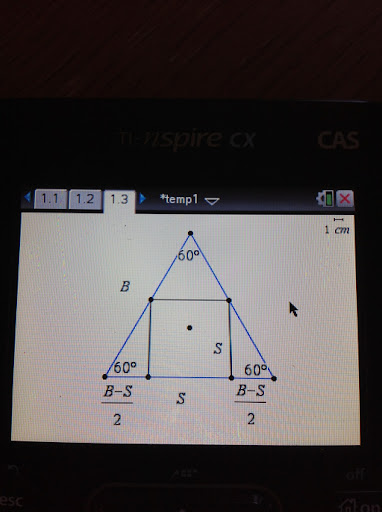Known: An equilateral triangle with sides of length B.
Goal: The length of the inscribed square, S.

Observe, by inspection, that the inscribed square is the largest when one of it's sides is touching the triangle's base. This allows for the largest S possible.

The measurement of the base is (B - S)/2 + S + (B - S)/2 = B.

We can find S by working with one of the two "mini right triangles" formed at the bottom of the triangle. A diagram of one of the "mini right triangles" is shown below: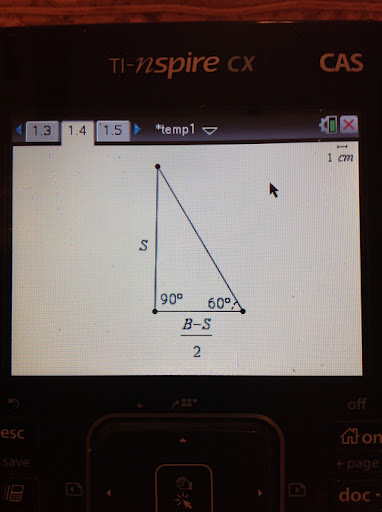Using trigonometry:

tan 60° = S / ((B - S) / 2)
√3 = (2S) / (B - S)
B √3 - S √3 = 2S

S = (B √3) / (2 + √3)

which is the desired result.

Largest Circle in an Equilateral TriangleI admit I have some difficulty with this one. In order for the circle to fit, the following have to be true:

1. The area of the triangle must be greater than the area of the circle.
2. The length of the triangle's side must be greater than twice the circle's radius.
3. The height of the triangle must be greater than twice the circle's radius.

Even obeying these rules did not guarantee an answer.

For me this is an open question. This may be one where numerical methods must be used. Any ideas, as always, are welcome.

Until then,

Eddie

This blog is property of Edward Shore. © 2012

### Casio fx-CG50: Sparse Matrix Builder

Casio fx-CG50: Sparse Matrix Builder Introduction The programs can create a sparse matrix, a matrix where most of the entries have zero valu...